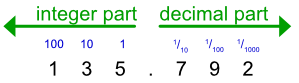Decimals

## Decimals

A decimal number has a decimal point.  Everything to the left of the decimal point is the integer part of the number, and everything to the right of the decimal point is the decimal part.

The integer part of the number is divided up into units, 10s, 100s and 1000s (as for integers).

The decimal part is divided into frac(1)(10), frac(1)(100), frac(1)(1000), with each new column (working to the right) being 10 times smaller than the previous column.In the example above, 135.792 is made up from:

1 is 1 x 100 = 100

3 is 3 x 10 = 30

5 has a value of 5

(decimal point)

7 is 7 x frac(1)(10) = 0.7 (frac(7)(10) as a fraction)

9 is 9 x frac(1)(100) = 0.09 (frac(9)(100))

2 is 2 x frac(1)(1000) = 0.002 (frac(2)(1000), or frac(1)(500))

The number of digits to the right of the decimal point is the number of decimal places. In the example above, the number has three decimal places.

## Example 1

What is the value of the digit 3 in 48.635? Show the answer as a fraction.

The three is in the hundredths column. 3 x frac(1)(100) = frac(3)(100).

Answer: frac(3)(100)

## Example 2

Which is the larger number, 23.65 or 23.592?

Working from the left, compare the digits by column.

Both have the same integer part.

Comparing the 1/10 column, the 6 on the first number is higher than the 5 on the second number, even though the second number has more places after the decimal point.